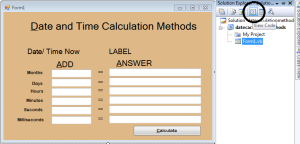# How to Create a Method for Calculating the Date and Time in VB.Net

0
1687

This tutorial is all about How to Create a Method for Calculating the Date and Time in VB.Net.
In this tutorial, I’m going to teach you how to calculate the date and time of the present date in VB.Net. This will help you calculate the months, days, hour, minutes, seconds and milliseconds to the present date. Then, the exact time will appear in each fields accurately.

So, let’s begin:
First, open the Visual Basic, create a new Windows Form Application and make the Form just like this.After doing that, go to the solution explorer and click the view code.In the code view, create a method for the calculation of the months, day and time.
[vbnet] Private Sub CalculatingMethods()

‘INITIALIZE THE INSTANCE METHODS OF THE DATETTIME TYPE.
Try
Dim date_Now As DateTime = DateAndTime.Now

lblOriginalDate.Text = date_Now

Catch ex As Exception
MsgBox(Me.Text, ex.Message)
End Try
End Sub
[/vbnet] After creating a method, you have to set the initial value of the TextBox and the method that you have created to fire it on the first load.
[vbnet] Private Sub Form1_Load(ByVal sender As System.Object, ByVal e As System.EventArgs) Handles MyBase.Load
‘SET THE INITIAL VALUE IN THE TEXTBOX
For Each txt As Control In Me.Controls
If txt.GetType Is GetType(TextBox) Then
txt.Text = 7
End If
Next
‘SET THE METHOD TO CALCULATE IN THE FIRST LOAD
CalculatingMethods()
End Sub
[/vbnet] And now, you have to set the methods that you have created in the `click` events handler of a “Calculate” Button.
[vbnet>
Private Sub Button1_Click(ByVal sender As System.Object, ByVal e As System.EventArgs) Handles Button1.Click
‘CALL THE METHODS TO CALCULATE EVERYTIME THE BUTTON IS CLICKED
Calculation_Methods()
End Sub
[/vbnet] Download the complete source code and run it on you computer.